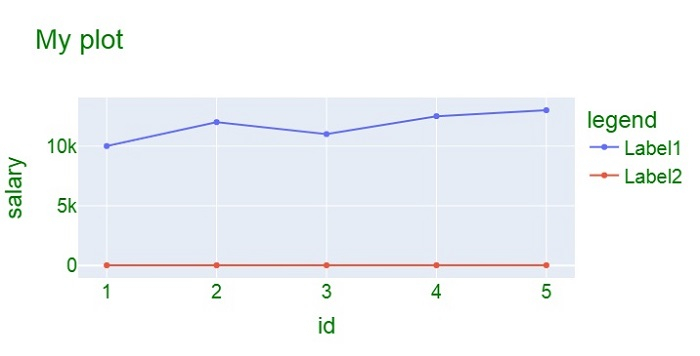# Python Plotly ŌĆō How to change variable/label names for the legend in a line chart?

Plotly is an open-source, interactive, and browser-based charting library for Python. Python users can use Plotly to generate different types of charts including scientific charts, 3D graphs, statistical charts, financial charts, etc.

In this tutorial, we will show how you can use Plotly to change the variable and label names for the legend in a line chart. Here we will use the plotly.graph_objects module to generate figures. It contains a lot of methods to customize the charts and render them into HTML format.

Follow the steps given below to generate variable or label names for the legend.

Step 1

Import the plotly.graphs_objs module and alias as go. Then, use the Figure() method to create a figure.

import plotly.graphs_objs as go
fig = go.Figure()


Step 2

Create a dictionary

data = {
'id':[1,2,3,4,5],
'salary':[10000,12000,11000,12500,13000],
'Age':[21,22,23,24,25]
}


Step 3

Use the add_trace() method to create traces for two scatter plots.

fig.add_trace(go.Scatter(
x=data['id'],
y=data['salary'],
name="Label1"
))
x=data['id'],
y=data['Age'],
name="Label2"
))


Step 4

Use the update_layout() method to change the X and Y-axis title and legend title. Update the layout for the X-axis and Y-axis and also set the legend title with the dict coordinates.

fig.update_layout(
title="My plot",
xaxis_title="id",
yaxis_title="salary",
legend_title="legend",
font=dict(family="Arial", size=20, color="green")
)


## Example

The complete code to change variable/label names for the legend is as follows ŌłÆ

import plotly.graph_objs as go
fig = go.Figure()
data = {
'id':[1,2,3,4,5],
'salary':[10000,12000,11000,12500,13000],
'Age':[21,22,23,24,25]
}

x=data['id'],
y=data['salary'],
name="Label1")
)

x=data['id'],
y=data['Age'],
name="Label2")
)

fig.update_layout(
title="My plot",
xaxis_title="id",
yaxis_title="salary",
legend_title="legend",
font=dict(
family="Arial",
size=20,
color="green"
)
)
fig.show()


## Output

It will show the following output on the browser ŌłÆ